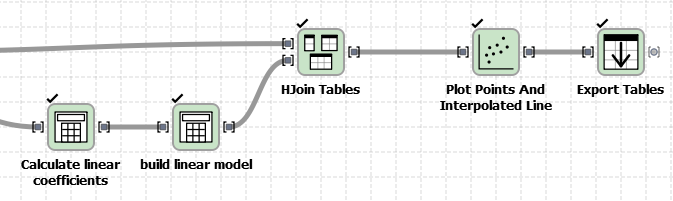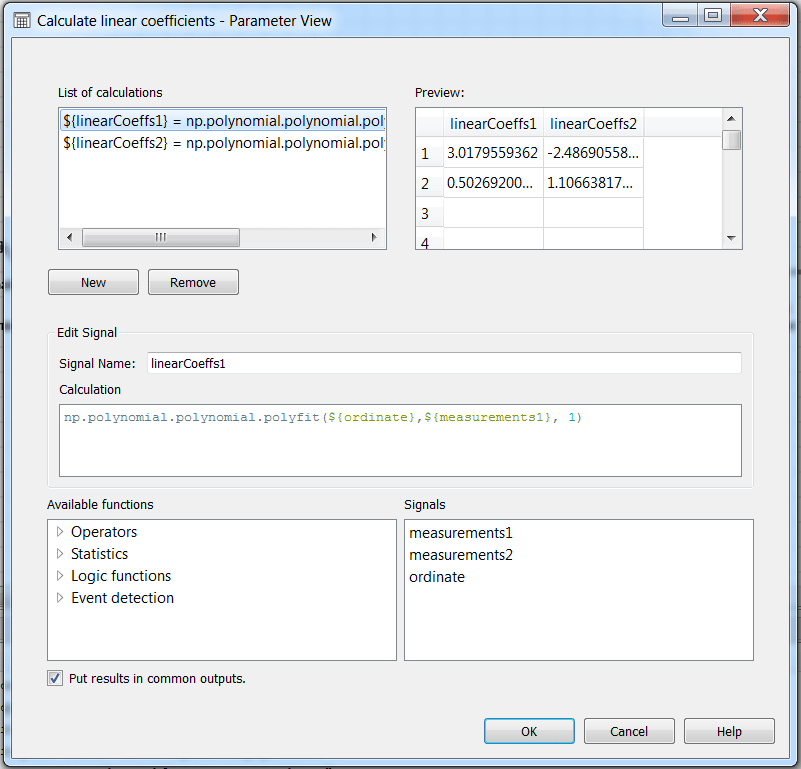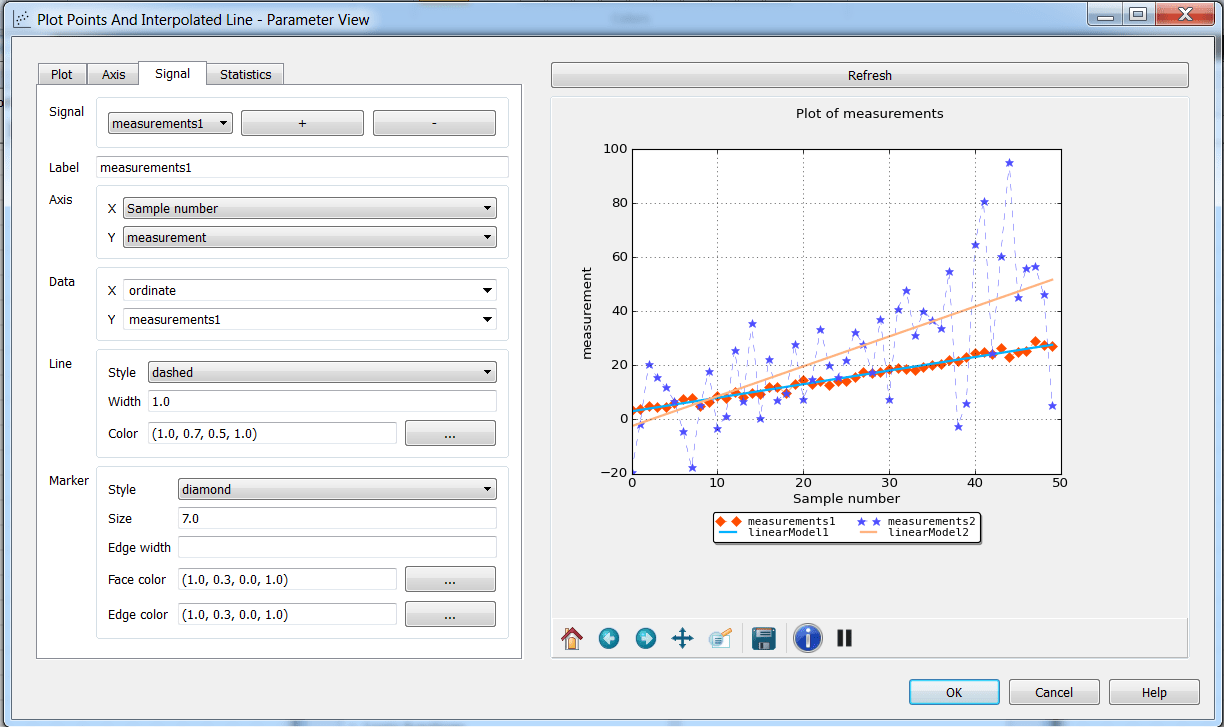Basic Statistics with Sympathy - Part 3: Make & Plot a simple regression with Sympathy - Combine | Combine

In our previous post, we briefly explored the plotting capabilities built in Sympathy, and also the enormous flexibility that the calculator node brings into our flow. Today we will use those two nodes to build and plot one of the simplest, most widely used models of data: a linear regression.

Our flow will simply have four parts:

1. A data capturing/manipulation part (that we use to obtain/import/filter the measurements and sample number / time information),
2. A part for obtaining the model coefficients
3. A part for generating a plot of the model together with the input data
4. A part for exporting the data and plots.

In the following figure, parts 2-4 are visible.For some help on how to do part 1, you can read here.

Regarding part 2, we will use the calculator node. More specifically in the flow in here, is the node in the leftmost bottom part that says “Calculate linear coefficients”. Our configuration screen looks as follows:The two input signals have the same size and were generated with the same time axis. As you can see, we are using polyfit in numpy for obtaining the model coefficients in each signal (see numpy documentation). This means that if you know your data can be better fit to another polynomial, you could request a different degree for the coefficients. In this example, for the two measurements, we have requested a first degree. Measurement 1 is more or less well behaved, while Measurement 2 is much noisier.

Now, the output of this calculation operation will be the two coefficients, ordered by the degree of the coefficient, i.e. if the model is mX^1 + bX^0, then we will have that linearCoeffs1 = b and linearCoeffs1 = m.

With this in mind, we can go to the configuration of the next calculator node (the one that says build linear model) in order to generate the data for plotting the model:So, since our linear model is Y = mX+b, then we generate a numpy array taking m and b from the linearCoeffs that we would like to plot, and we do that over the length of the original measurements (in this example it was 50 samples). In this way, the output signal from the plottable model is as long as the input data, and we can easily apply “Hjoin Tables”, in order to have all the information we need to plot it.

After joining the original data with the models, we can go to the configuration of the plot node, and put together the model and the input data in the same canvas. You can play with the colors, the types of lines and markers, add a grid, place proper names, and even add the mean of each signal in the label (as the featured image in this post).The final step is exporting. A common use of the plotter in sympathy is as an inspection tool for signals that we have in our data, in that way we may notice if we need to filter the data a bit better or if the signals are behaving as expected. Then, to export the plots in the plotting node, we feed  the signal into an exporter, and we choose the type of file we want to export the graphic in “plot output extension”. We can choose between png, pdf, and a couple more. This will export both the data and the plot.

And you are set! I hope you have some nice holidays. I will do, for sure!